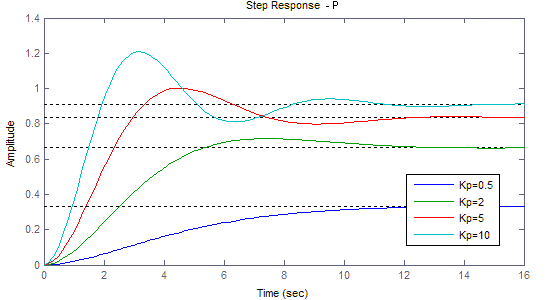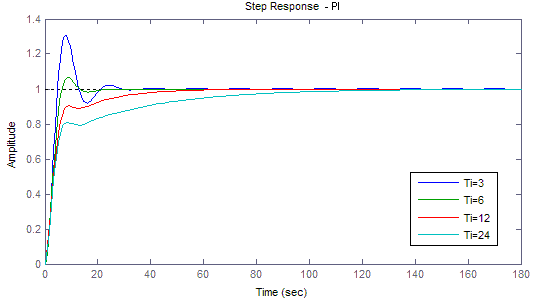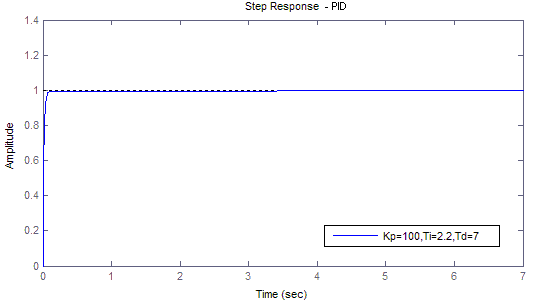# 用MATLAB对PID控制做简单的仿真

PID控制是目前工程上应用最广的一种控制方法，其结构简单，且不依赖被控对象模型，控制所需的信息量也很少，因而易于工程实现，同时也可获得较好的控制效果。

PID控制是将误差信号e(t)的比例(P)，积分(I)和微分(D)通过线性组合构成控制量进行控制，其输出信号为：

$u(t) = {K_P}[e(t) + \frac{1}{{{T_I}}}\int_0^t {e(t)dt + {T_D}\frac{{de(t)}}{{dt}}} ]$

1. 建立二阶负反馈控制系统，其开环传递函数为：

${G_O}(s) = \frac{1}{{(2s + 1)(5s + 1)}}$

2. 比例控制，输出与输入偏差成比例，即直接将误差信号放大或缩小。比例控制的传递函数为：

${G_C}(s) = {K_P}$3. 微分控制，输出与输入偏差的微分成比例，即与偏差的变化速度成比例。微分控制（与比例控制同时使用）的传递函数为：

${G_C}(s) = {K_P}(1 + {T_D}s)$4. 积分控制，输出与输入偏差的积分成比例，即与误差的积累成比例。积分控制（与比例控制同时使用）的传递函数为：

${G_C}(s) = {K_P}(1 + \frac{1}{{{T_I}}} \cdot \frac{1}{s})$5. 比例积分微分控制，即PID控制的传递函数为：

${G_C}(s) = {K_P}(1 + \frac{1}{{{T_I}}} \cdot \frac{1}{s} + {T_D}s)$PID控制通过积分作用消除误差，而微分控制可缩小超调量、加快系统响应，是综合了PI控制和PD控制长处并去除其短处的控制。